# Understanding bind

This post is the second in a series. In the previous post, I described some of the core functions for lifting a value from a normal world to an elevated world.

In this post, we'll look at "world-crossing" functions, and how they can be tamed with the `bind` function.

## Series contents

Here's a list of shortcuts to the various functions mentioned in this series:

• Part 1: Lifting to the elevated world
• Part 2: How to compose world-crossing functions
• Part 3: Using the core functions in practice
• Part 4: Mixing lists and elevated values
• Part 5: A real-world example that uses all the techniques
• Part 6: Designing your own elevated world
• Part 7: Summary

## The `bind` function

Common Names: `bind`, `flatMap`, `andThen`, `collect`, `SelectMany`

Common Operators: `>>=` (left to right), `=<<` (right to left )

What it does: Allows you to compose world-crossing ("monadic") functions

Signature: `(a->E<b>) -> E<a> -> E<b>`. Alternatively with the parameters reversed: `E<a> -> (a->E<b>) -> E<b>`

### Description

We frequently have to deal with functions that cross between the normal world and the elevated world.

For example: a function that parses a `string` to an `int` might return an `Option<int>` rather than a normal `int`, a function that reads lines from a file might return `IEnumerable<string>`, a function that fetches a web page might return `Async<string>`, and so on.

These kinds of "world-crossing" functions are recognizable by their signature `a -> E<b>`; their input is in the normal world but their output is in the elevated world. Unfortunately, this means that these kinds of functions cannot be linked together using standard composition.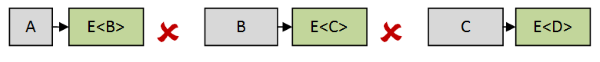What "bind" does is transform a world-crossing function (commonly known as a "monadic function") into a lifted function `E<a> -> E<b>`.The benefit of doing this is that the resulting lifted functions live purely in the elevated world, and so can be combined easily by composition.

For example, a function of type `a -> E<b>` cannot be directly composed with a function of type `b -> E<c>`, but after `bind` is used, the second function becomes of type `E<b> -> E<c>`, which can be composed.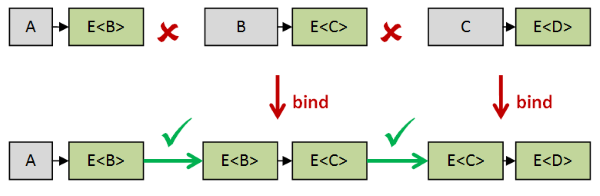In this way, `bind` lets us chain together any number of monadic functions.

### Alternative interpretation

An alternative interpretation of `bind` is that it is a two parameter function that takes a elevated value (`E<a>`) and a "monadic function" (`a -> E<b>`), and returns a new elevated value (`E<b>`) generated by "unwrapping" the value inside the input, and running the function `a -> E<b>` against it. Of course, the "unwrapping" metaphor does not work for every elevated world, but still it can often be useful to think of it this way.### Implementation examples

Here are some examples of defining `bind` for two different types in F#:

``````module Option =

// The bind function for Options
let bind f xOpt =
match xOpt with
| Some x -> f x
| _ -> None
// has type : ('a -> 'b option) -> 'a option -> 'b option

module List =

// The bind function for lists
let bindList (f: 'a->'b list) (xList: 'a list)  =
[ for x in xList do
for y in f x do
yield y ]
// has type : ('a -> 'b list) -> 'a list -> 'b list
``````

Notes:

• Of course, in these two particular cases, the functions already exist in F#, called `Option.bind` and `List.collect`.
• For `List.bind` I'm cheating again and using `for..in..do`, but I think that this particular implementation shows clearly how bind works with lists. There is a purer recursive implementation, but I won't show that here.

### Usage example

As explained at the beginning of this section, `bind` can be used to compose cross-world functions. Let's see how this works in practice with a simple example.

First let's say we have a function that parses certain `string`s into `int`s. Here's a very simple implementation:

``````let parseInt str =
match str with
| "-1" -> Some -1
| "0" -> Some 0
| "1" -> Some 1
| "2" -> Some 2
// etc
| _ -> None

// signature is string -> int option
``````

Sometimes it returns a int, sometimes not. So the signature is `string -> int option` -- a cross-world function.

And let's say we have another function that takes an `int` as input and returns a `OrderQty` type:

``````type OrderQty = OrderQty of int

let toOrderQty qty =
if qty >= 1 then
Some (OrderQty qty)
else
// only positive numbers allowed
None

// signature is int -> OrderQty option
``````

Again, it might not return an `OrderQty` if the input is not positive. The signature is therefore `int -> OrderQty option` -- another cross-world function.

Now, how can we create a function that starts with an string and returns an `OrderQty` in one step?

The output of `parseInt` cannot be fed directly into `toOrderQty`, so this is where `bind` comes to the rescue!

Doing `Option.bind toOrderQty` lifts it to a `int option -> OrderQty option` function and so the output of `parseInt` can be used as input, just as we need.

``````let parseOrderQty str =
parseInt str
|> Option.bind toOrderQty
// signature is string -> OrderQty option
``````

The signature of our new `parseOrderQty` is `string -> OrderQty option`, yet another cross-world function. So if we want to do something with the `OrderQty` that is output we may well have to use `bind` again on the next function in the chain.

### Infix version of bind

As with `apply`, using the named `bind` function can be awkward, so it is common to create an infix version, typically called `>>=` (for left to right data flow) or `=<<` (for right to left data flow) .

With this in place you can write an alternative version of `parseOrderQty` like this:

``````let parseOrderQty_alt str =
str |> parseInt >>= toOrderQty
``````

You can see that `>>=` performs the same kind of role as pipe (`|>`) does, except that it works to pipe "elevated" values into cross-world functions.

### Bind as a "programmable semicolon"

Bind can be used to chain any number of functions or expressions together, so you often see code looking something like this:

``````expression1 >>=
expression2 >>=
expression3 >>=
expression4
``````

This is not too different from how an imperative program might look if you replace the `>>=` with a `;`:

``````statement1;
statement2;
statement3;
statement4;
``````

Because of this, `bind` is sometimes called a "programmable semicolon".

### Language support for bind/return

Most functional programming languages have some kind of syntax support for `bind` that lets you avoid having to write a series of continuations or use explicit binds.

In F# it is (one component) of computation expressions, so the following explicit chaining of `bind`:

``````initialExpression >>= (fun x ->
expressionUsingX  >>= (fun y ->
expressionUsingY  >>= (fun z ->
x+y+z )))             // return
``````

becomes implicit, using `let!` syntax:

``````elevated {
let! x = initialExpression
let! y = expressionUsingX x
let! z = expressionUsingY y
return x+y+z }
``````

In Haskell, the equivalent is the "do notation":

``````do
x <- initialExpression
y <- expressionUsingX x
z <- expressionUsingY y
return x+y+z
``````

And in Scala, the equivalent is the "for comprehension":

``````for {
x <- initialExpression
y <- expressionUsingX(x)
z <- expressionUsingY(y)
} yield {
x+y+z
}
``````

It's important to emphasize that you do not have to use the special syntax when using bind/return. You can always use `bind` or `>>=` in the same way as any other function.

### Bind vs. Apply vs. Map

The combination of `bind` and `return` are considered even more powerful than `apply` and `return`, because if you have `bind` and `return`, you can construct `map` and `apply` from them, but not vice versa.

Here's how bind can be used to emulate `map`, for example:

• First, you construct a world-crossing function from a normal function by applying `return` to the output.
• Next, convert this world-crossing function into a lifted function using `bind`. This gives you the same result as if you had simply done `map` in the first place.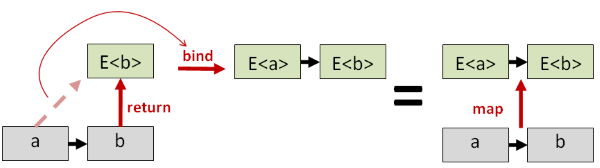Similarly, `bind` can emulate `apply`. Here is how `map` and `apply` can be defined using `bind` and `return` for Options in F#:

``````// map defined in terms of bind and return (Some)
let map f =
Option.bind (f >> Some)

// apply defined in terms of bind and return (Some)
let apply fOpt xOpt =
fOpt |> Option.bind (fun f ->
let map = Option.bind (f >> Some)
map xOpt)
``````

At this point, people often ask "why should I use `apply` instead of `bind` when `bind` is more powerful?"

The answer is that just because `apply` can be emulated by `bind`, doesn't mean it should be. For example, it is possible to implement `apply` in a way that cannot be emulated by a `bind` implementation.

In fact, using `apply` ("applicative style") or `bind` ("monadic style") can have a profound effect on how your program works! We'll discuss these two approaches in more detail in part 3 of this post.

### The properties of a correct bind/return implementation

As with `map`, and as with `apply`/`return`, a correct implementation of the `bind`/`return` pair should have some properties that are true no matter what elevated world we are working with.

There are three so-called "Monad Laws", and one way of defining a Monad (in the programming sense) is to say that it consists of three things: a generic type constructor `E<T>` plus a pair of functions (`bind` and `return`) that obey the monad laws. This is not the only way to define a monad, and mathematicians typically use a slightly different definition, but this one is most useful to programmers.

Just as with the the Functor and Applicative laws we saw earlier, these laws are quite sensible.

First, note that `return` function is itself a cross-world function: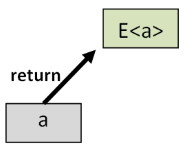That means that we can use `bind` to lift it into a function in the elevated world. And what does this lifted function do? Hopefully, nothing! It should just return its input.

So that is exactly the first monad law: it says that this lifted function must be the same as the `id` function in the elevated world.The second law is similar but with `bind` and `return` reversed. Say that we have a normal value `a` and cross-world function `f` that turns an `a` into a `E<b>`.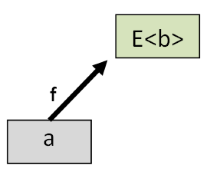Let's lift both of them to the elevated world, using `bind` on `f` and `return` on `a`.Now if we apply the elevated version of `f` to the elevated verson of `a` we get some value `E<b>`.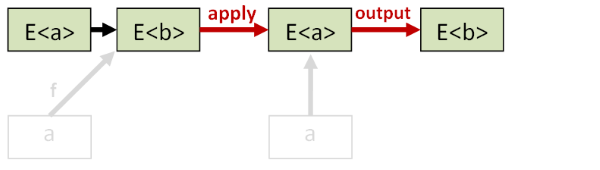On the other hand if we apply the normal version of `f` to the normal verson of `a` we also get some value `E<b>`.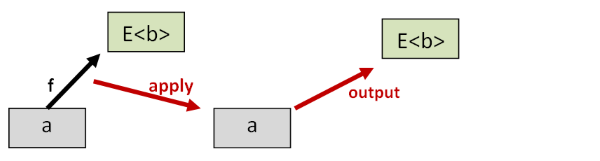The second monad law says that these two elevated values (`E<b>`) should be the same. In other words, all this binding and returning should not distort the data.

In the normal world, function composition is associative. For example, we could pipe a value into a function `f` and then take that result and pipe it into another function `g`. Alternatively, we can compose `f` and `g` first into a single function and then pipe `a` into it.

``````let groupFromTheLeft = (a |> f) |> g
let groupFromTheRight = a |> (f >> g)
``````

In the normal world, we expect both of these alternatives to give the same answer.

The third monad law says that, after using `bind` and `return`, the grouping doesn't matter either. The two examples below correspond to the examples above:

``````let groupFromTheLeft = (a >>= f) >>= g
let groupFromTheRight = a >>= (fun x -> f x >>= g)
``````

And again, we expect both of these to give the same answer.

If you look at the definition above, a monad has a type constructor (a.k.a "generic type") and two functions and a set of properties that must be satisfied.

The `List` data type is therefore just one component of a monad, as is the `Option` data type. `List` and `Option`, by themselves, are not monads.

It might be better to think of a monad as a transformation, so that the "List monad" is the transformation that converts the normal world to the elevated "List world", and the "Option monad" is the transformation that converts the normal world to the elevated "Option world".

I think this is where a lot of the confusion comes in. The word "List" can mean many different things:

1. A concrete type or data structure such as `List<int>`.
2. A type constructor (generic type): `List<T>`.
3. A type constructor and some operations, such as a `List` class or module.
4. A type constructor and some operations and the operations satisfy the monad laws.

Only the last one is a monad! The other meanings are valid but contribute to the confusion.

Also the last two cases are hard to tell apart by looking at the code. Unfortunately, there have been cases where implementations did not satisfy the monad laws. Just because it's a "Monad" doesn't mean that it's a monad.

Personally, I try to avoid using the word "monad" on this site and focus on the `bind` function instead, as part of a toolkit of functions for solving problems rather than an abstract concept.

Do ask: Do I have useful bind and return functions? And are they implemented correctly?

## Summary

We now have a set of four core functions: `map`, `return`, `apply`, and `bind`, and I hope that you are clear on what each one does.

But there are some questions that have not been addressed yet, such as "why should I choose `apply` instead of `bind`?", or "how can I deal with multiple elevated worlds at the same time?"

In the next post we'll address these questions and demonstrate how to use the toolset with a series of practical examples.

UPDATE: Fixed error in monad laws pointed out by @joseanpg. Thanks!